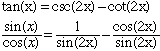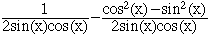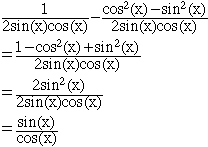Sender: Rhiannon Subject: Trig identity crisis I have tried many times to find the answer to these problems but I can't I am in grade 12 tan(x)=csc2(x)-cot2(x) cos(x)/csc(x)-2sin(x)=tan(x)/1-tan(x) cos(x)[ tan2(x)1-1]/cos2(x)+sin2(x)=sec(x) Thank you Hi Rhiannon, I'll show you how I attack these problems by doing problem 1. First I would transform all the trig functions to sines and cosines and rewrite the identity.Next work on the right side. Use the double angle formulas to replace sin(2x) and cos(2x) with expressions involving sin(x) and cos(x)Now simplify using the fact that sin2(x) + cos2(x) = 1.Hence the right side and left side are equal. Cheers, Harley Go to Math Central